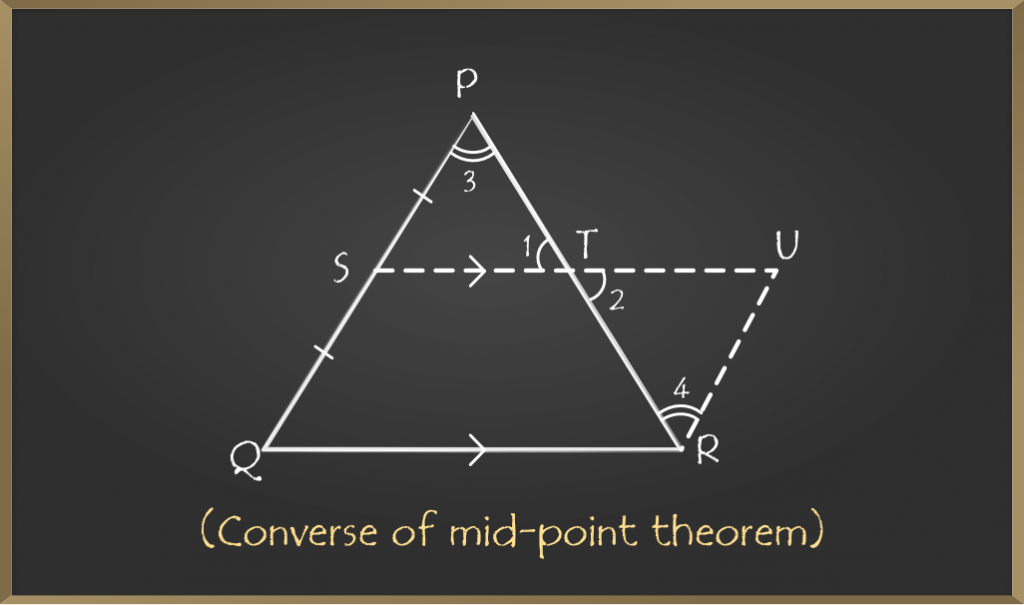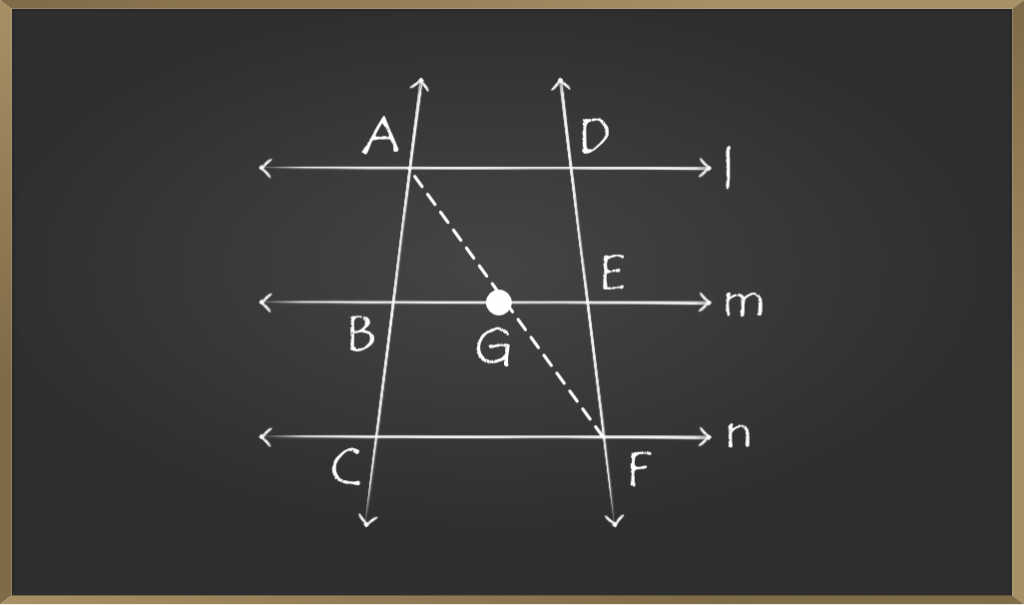# Mid Point Theorem – Quadrilaterals | Class 9 Maths

• Last Updated : 28 Oct, 2020

Geometry is an important part of mathematics that deals with different shapes and figures. Triangles are an important part of geometry and the mid-point theorem points towards mid points of the triangle.

## What is Mid-Point Theorem?

This theorem states that” The line segment joining mid-points of two sides of a triangle is parallel to the third side of the triangle and is half of it”## Proof of Mid-Point Theorem

A triangle ABC in which D is the mid-point of AB and E is the mid-point of AC.

To Prove: DE ∥ BC and DE = 1/2(BC)

### Construction

Extend the line segment joining points D and E to F such that DE = EF and join CF.

### Proof

In ∆AED and ∆CEF

DE = EF (construction)

∠1 = ∠2 (vertically opposite angles)

AE = CE (E is the mid-point)

△AED ≅ △CEF by SAS criteria

Therefore,

∠3 =∠4 (c.p.c.t)

But these are alternate interior angles.

So, AB ∥ CF

But AD = DB (D is the mid-point)

Therefore, BD = CF

In BCFD

BD∥ CF (as AB ∥ CF)

BD = CF

BCFD is a parallelogram as one pair of opposite sides is parallel and equal.

Therefore,

DF∥ BC (opposite sides of parallelogram)

DF = BC (opposite sides of parallelogram)

As DF∥ BC, DE∥ BC and DF = BC

But DE = EF

So, DF = 2(DE)

2(DE) = BC

DE = 1/2(BC)

Hence, proved that the line joining mid-points of two sides of the triangle is parallel to the third side and is half of it.

## What is the Converse of Mid-Point Theorem?

The line drawn through the mid-point of one side of a triangle parallel to the base of a triangle bisects the third side of the triangle.## Proof of the Theorem

In triangle PQR, S is the mid-point of PQ and ST ∥ QR

To Prove: T is the mid-point of PR.

### Construction

Draw a line through R parallel to PQ and extend ST to U.

### Proof

ST∥ QR(given)

So, SU∥ QR

PQ∥ RU (construction)

Therefore, SURQ is a parallelogram.

SQ = RU(Opposite sides of parallelogram)

But SQ = PS (S is the mid-point of PQ)

Therefore, RU = PS

In △PST and △RUT

∠1 =∠2(vertically opposite angles)

∠3 =∠4(alternate angles)

PS = RU(proved above)

△PST ≅ △RUT by AAS criteria

Therefore, PT = RT

T is the mid-point of PR.

### Sample Problems on Mid Point Theorem

Problem 1: l, m, and n are three parallel lines. p and q are two transversals intersecting parallel lines at A, B, C, D, E, and F as shown in the figure.If AB:BC = 1:1, find the ratio of DE : EF.Given: AB:BC=1:1

To find: DE:EF

Construction: Join AF such that it intersects line m at G.

In △ACF

AB = BC(1:1 ratio)

BG∥ CF(as m∥n)

Therefore, by converse of mid-point theorem G is the midpoint of AF(AG = GF)

Now, in △AFD

AG = GF(proved above)

Therefore, by converse of mid-point theorem E is the mid-point of DF(FE = DE)

So, DE:EF = 1:1(as they are equal)

Problem 2: In the figure given below L, M and N are mid-points of side PQ, QR, and PR respectively of triangle PQR.

If PQ = 8cm, QR = 9cm and PR = 6cm. Find the perimeter of the triangle formed by joining L, M, and N.Solution: As L and N are mid-points

By mid-point theorem

LN ∥ QR and LN = 1/2 * (QR)

LN = 1/2 × 9 = 4.5cm

Similarly, LM = 1/2 * (PR) = 1/2×(6) = 3cm

Similarly, MN = 1/2 * (PQ) = 1/2 × (8) = 4cm

Therefore, the perimeter of △LMN is LM + MN + LN

= 3 + 4 + 4.5

= 11.5cm

Perimeter is 11.5cm

Result: Perimeter of the triangle formed by joining mid-points of the side of the triangle is half of the triangle.

My Personal Notes arrow_drop_up